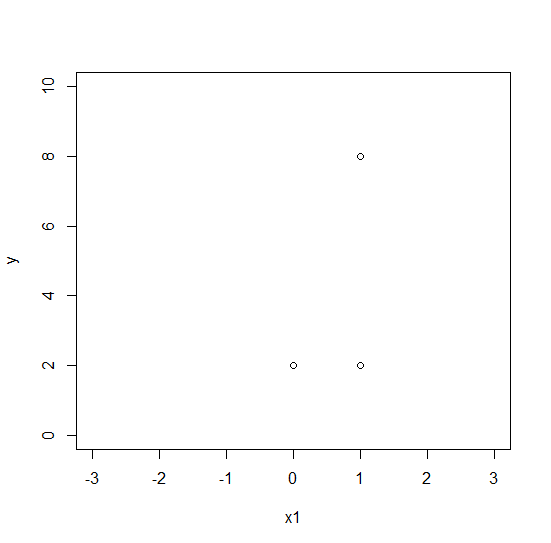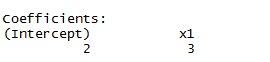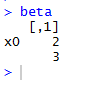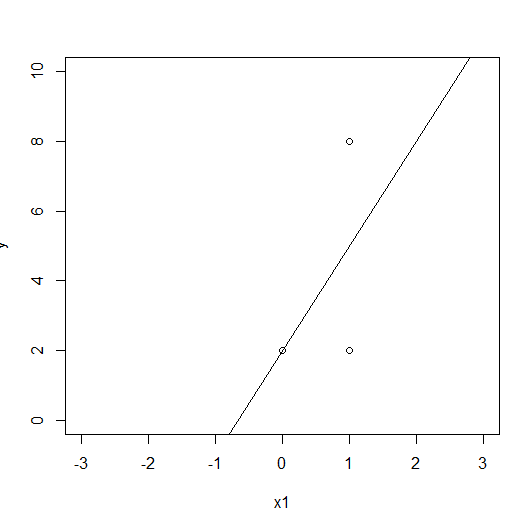## Populating a Time Dimension

A ready-made script that I have modified to create and populate a Kimball Time dimension.

This script will create a time dimension and populate it with different levels of granularity: second, minute, hour.

--Create the time dim table

CREATE TABLE [dbo].[DimTime](
[TimeKey] [int] NOT NULL,
[TimeAltKey] [int] NOT NULL,
[Time] [varchar](8) NOT NULL,
[TimeMinutes] [varchar](5) NULL,
[TimeHours] [varchar](2) NULL,
[HourNumber] [tinyint] NOT NULL,
[MinuteNumber] [tinyint] NOT NULL,
[SecondNumber] [tinyint] NOT NULL,
[TimeInSecond] [int] NOT NULL,
CONSTRAINT [PK_DimTime] PRIMARY KEY CLUSTERED
(
[TimeKey] ASC
)
)

--Script to populate the time dimension
CREATE PROCEDURE [dbo].[p_InsertDimTime]
as
BEGIN
--Specify Total Number of Hours You need to fill in Time Dimension
DECLARE @Size INTEGER
--iF @Size=32 THEN This will Fill values Upto 32:59 hr in Time Dimension
Set @Size=23
DECLARE @hour INTEGER
DECLARE @minute INTEGER
DECLARE @second INTEGER
DECLARE @TimeKey INTEGER
DECLARE @TimeAltKey INTEGER
DECLARE @TimeInSeconds INTEGER
DECLARE @Time varchar(25)
DECLARE @hourTemp varchar(4)
DECLARE @minTemp varchar(4)
DECLARE @secTemp varchar(4)
SET @hour = 0
SET @minute = 0
SET @second = 0
SET @TimeKey = 0
SET @TimeAltKey = 0
WHILE(@hour<= @Size )
BEGIN
if (@hour <10 )
begin
set @hourTemp = '0' + cast( @hour as varchar(10))
end
else
begin
set @hourTemp = @hour
end
WHILE(@minute <= 59)
BEGIN
WHILE(@second <= 59)
BEGIN
set @TimeAltKey = @hour *10000 +@minute*100 +@second
set @TimeInSeconds =@hour * 3600 + @minute *60 +@second
If @minute <10
begin
set @minTemp = '0' + cast ( @minute as varchar(10) )
end
else
begin
set @minTemp = @minute
end
if @second Continue reading "Populating a Time Dimension"

/

## Implement Linear Regression in R (single variable)

Linear regression is probably one of the most well known and used algorithms in  machine learning.

In this post, I will discuss about how to implement linear regression step by step in R.

Let’s first create our dataset in R that contains only one variable “x1” and the variable that we want to predict “y”.

#Linear regression single variable

data <- data.frame(x1=c(0, 1, 1), y = c(2, 2, 8))

#ScatterPlot

plot(data, xlab=’x1′, ylab=’y’,xlim=c(-3,3), ylim=c(0,10))We now have three points with coordinates: (0;2),(1;2),(1;8) and we want to dar the best fit line that will best represents our data on a scatter plot.

In the part 1 I will implement the different calculation step to get the best fine using some linear algebra, however, in R we don’t need to do the math as there’s already a bult-in function called “lm” which computes the linear regression calculation.

So, if you just want to use the linear regression function straight away and don’t go through the different step to implement a linear model you can skip the part 1 and go to the part 2. I’d still recommand to undertsand how the algorithm works than just using it.

Part 1: Linear regression (with linear algebra calculation)

In order to find the best fit line that minimizes the sum of the square differences between the left and right sides we’ll compute the normal equation:#Output vector y

y = c(2, 2, 8)

#Input vector x1

x1=c(0, 1, 1)

#Intercept vector (it is simply the value at which the fitted line crosses the y-axis)

x0<-rep(1,nrow(y))

#Let’s create my Y matrix

Y <- as.matrix(data$y) #Let’s create ny X matrix X <- as.matrix(cbind(x0,data$x1))

#Let’s compute the normal equation

beta = solve(t(X) %*% X) %*% (t(X) %*% Y)

The result of the normal equation is: 2*x0 +3*x1

The best fit line equation is : 3×1+2 (remember x0 is always 1)

With R we can use the lm function which will do the math for us:

fit <- lm(y~+x1)

We can compare in R if our variable fit and beta are equivalent.

fit:beta:Plot the best fit line:

abline(beta) or abline(fit)We now have our best fit line drwan on our scatterplot btu now we want to find the coefficient of determination, denoted R2.

In order to calculate the R squared we need to calculate the “baseline prediction”, the “residual sum of squares (RSS)” and the “Total Sum of Squares (SST)”.

Baseline prediction is just is the average value of our dependent variable.(2+2+8)/3 = 4

The mean can also be computed in R as follows :

baseline <- mean(y)  or beasline <- sum(y)/nrow(y)

Residual sum of squares (RSS) or (SSR/SSE)  is the sum of the squares of residuals (deviations predicted from actual empirical values of data). It is a measure of the difference between the data and an estimation model. A small RSS indicates a good fit of the model to the data.Let’s implement the RSS in R:

#We first get all our values for f(xi)

Ypredict<-predict(fit,data.frame(x1))

#Then we compute the squared difference between y and f(xi) (Ypredict)

RSS<- sum((y – Ypredict)^2) #which gives ((2 – 2)^2 + (2 – 5)^2 + (8 – 5)^2) = 18

Total Sum of Squares (SST) or (TSS) is a statistical method which evaluates the sum of the squared difference between the actual X and the mean of X, from the overall mean.SST<-sum((y-baseline)^2) #baseline is the average of  y

We can now calculate the R squared:RSquare<-1 – (RSS/ SST) #which gives 1 – (18/24)=0.25

Part2: Quick way (without linear algebra)

data <- data.frame(x1=c(0, 1, 1), y = c(2, 2, 8))

plot(data, xlab=’x1′, ylab=’y’,xlim=c(-3,3), ylim=c(0,10))

fit <- lm(y~+x1)

abline(fit)

str(summary(fit)) # Will return many information included the R squared

/